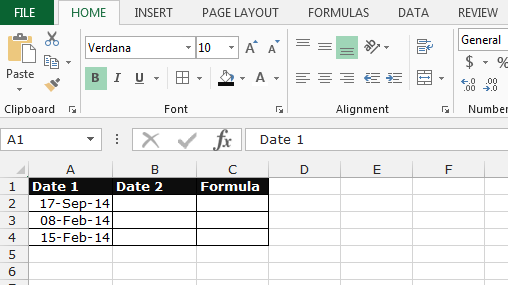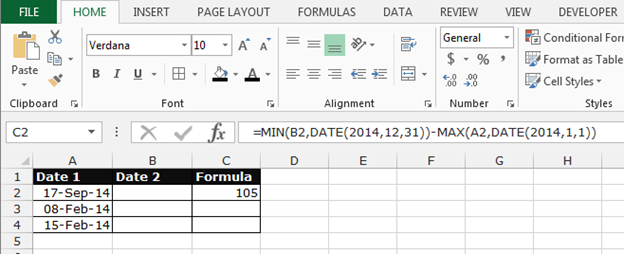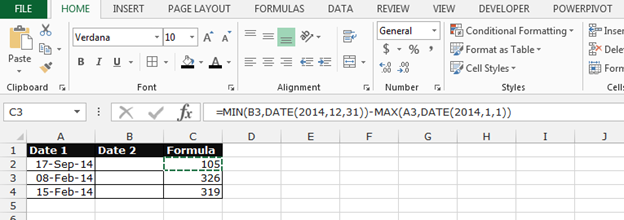# Avoiding Negative Values when Calculating the Number of Days between Two Dates in Microsoft Excel 2010

In this article you’ll learn, how to avoid negative values while calculating the number of days between two dates. We’ll use MIN, MAX and DATE function in Microsoft Excel.

• To solve this problem Date function will help to return the number that represent the date in Microsoft Excel date time code
• MAX function will be return the largest number in a set of values
• MIN function will return smallest number in a set of values

Let’s take an example,

We want to create a formula to calculate the number of days between each pair of dates in columns A & B, but the formula is never to return a negative value.Empty cells in column A are to be considered the first day of the year, and empty cells in column B are to be considered the last day of the year.

• Write the formula in cell C2
• =MIN(B2,DATE(2014,12,31))-MAX(A2,DATE(2014,1,1))
• Press Enter
• The function will return 105• Copy the same formula using Ctrl+C and paste in the range C3:C4 by usingIn this way, we can avoid the negative values while calculating the number of days between two dates in Microsoft Excel.We would love to hear from you, do let us know how we can improve, complement or innovate our work and make it better for you. Write us at info@exceltip.com.

1.